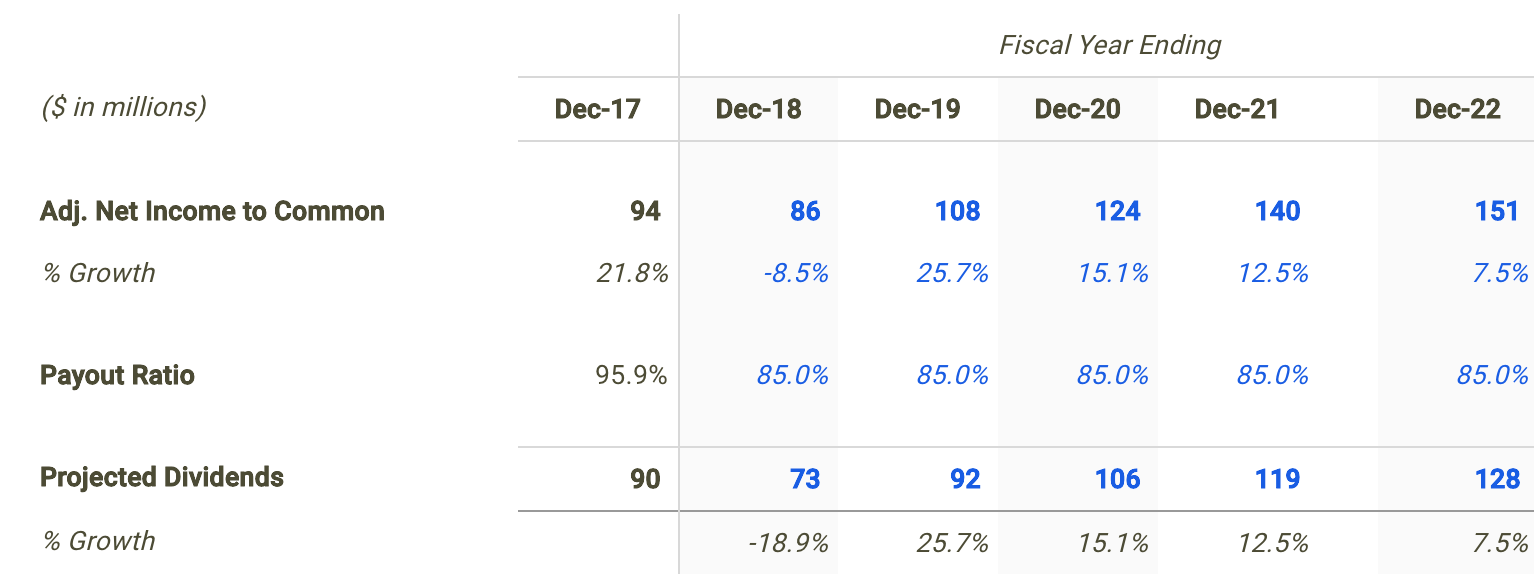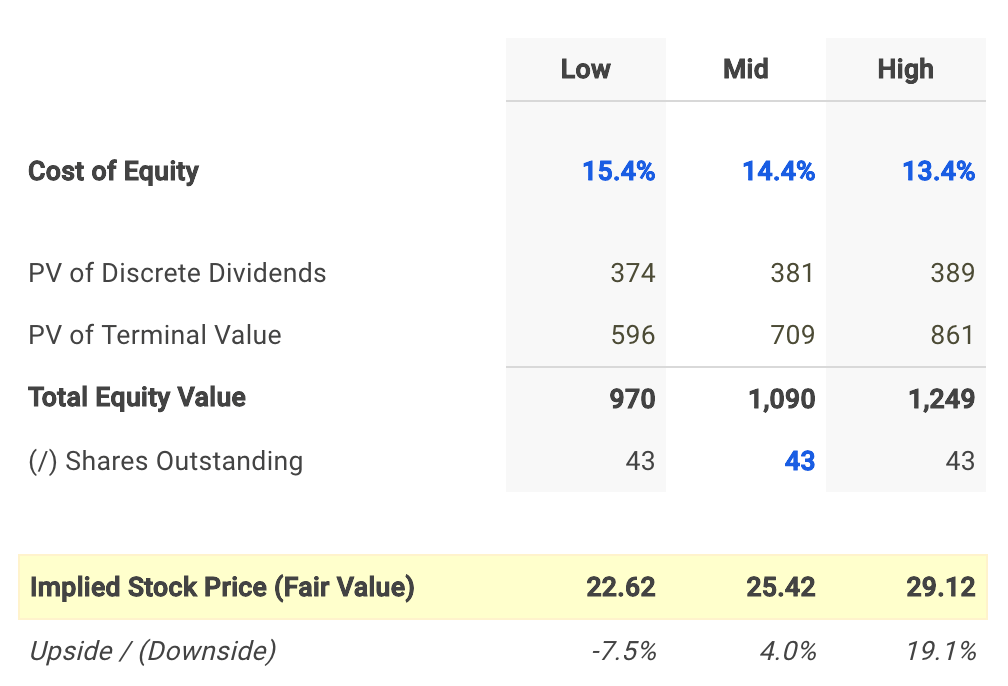GasLog Partners LP (NYSE: GLOP) has distributed 95.9% of its profits in the form of dividends over the last twelve months making the stock a prime candidate to be valued using a dividend discount analysis. Operating as an energy company paying a dividend yield of 8.5%, could the small-cap stock be trading at an attractive valuation? Let’s take a look at the future dividend potential of GasLog to see if there is any catalyst for a price change.

##### Application Of The Dividend Discount Model

The Dividend Discount Model (DDM) is a dividend-based valuation model that estimates the present value of a stock based on assumptions about its future dividend performance.

But before discussing the assumptions used in my dividend discount model for GasLog, it’s first helpful to determine if this is actually an appropriate technique to be used when estimating its fair value. Many analysts find it difficult when trying to figure out the correct valuation methodology for a given company or are biased towards one specific approach. This is often a mistake which can negatively impact investment decisions and result in trading losses or missed opportunities. No two companies are the same and every business consists of unique characteristics that may require you to adjust your analysis.

Understanding leverage trends is the first step when determining what valuation analyses are relevant for a given company. When a company’s leverage doesn’t fluctuate or is expected to remain stable over time, then an equity valuation model (e.g. equity DCF, DDM) will be the most appropriate valuation technique. The reason for this is because when leverage is stable, interest expense on debt can typically be projected with much more reliability.

How do we check if a company’s leverage has been fluctuating or is expected to do so? This isn’t always straightforward but checking recent debt ratio trends can be a good indicator. GasLog’s debt to equity ratio has been relatively consistent over last few years with a range spanning only 14.5%. This suggests that an equity valuation model is a suitable technique when valuing the company’s shares. Now does it make sense to use a dividend discount model knowing that an equity valuation technique is an appropriate methodology?

The next step is to determine if GasLog pays a dividend and if so, is its payout ratio relatively high (typically above 70%)? The table below provides this information in more detail.Source: GasLog dividend discount model

GasLog distributed a total of \$90 million in cash dividends to shareholders in its most recent fiscal year Dec-17 which represented a payout ratio of 95.9%. It appears that the company meets both criteria. Therefore, it is fitting to apply a dividend discount model when calculating the intrinsic value of GasLog.

##### Projecting The Future Dividends Of GasLog

The first step in building a dividend discount model is to forecast net income since forecasting dividends directly can be difficult. So let’s create a net income forecast for the next five years and use that as the basis for projecting future dividends.

Finbox.io applies consensus Wall Street estimates for the net income forecast when available. For the next fiscal year 2018, profits are expected to decrease -8.5%, then grow 25.7% in 2019 and rise 15.1% in 2020.Source: GasLog Projected Net Income Growth

I use the projections above to serve as the basis for my dividend projections. The next step is to forecast the company’s payout ratio. In my estimates shown in the table below, I select an 85.0% payout ratio in 2018 and hold it steady there throughout the remainder of my projection period.Source: GasLog dividend discount model

##### Calculating GasLog’s Intrinsic Value

Finally, we can now calculate GasLog’s intrinsic value by present valuing its forecasted dividends. Note that we apply the company’s cost of equity to discount the future dividends since these payments are made to common shareholders or equity owners. I used finbox.io’s Weighted Average Cost of Capital (WACC) model to help arrive at an estimate for the company’s cost of equity.

I determined a reasonable cost of equity for GasLog to be 14.4% at the midpoint. An updated cost of capital analysis using real-time data can be found at finbox.io’s GasLog WACC modelpage.My dividend forecast and cost of capital assumptions imply a fair value per share for GasLog of \$25.42, 4.0% above its current stock price of \$24.15. As a result, investors may conclude that they want to hold off on purchasing shares until the stock develops a wider margin of safety.

##### Conclusion: Dividends Support Stock Price

Finding the true value of a company can sometimes be difficult but determining an appropriate valuation methodology should not be. Knowing when and when not to use the dividend discount model will help in your investment decision making process.

But it is important to note that a dividend discount model will inherently undervalue a company’s stock. This is typically the result of the payout ratio assumption being less than 100% implying some cash leakage. In reality, this excess retained cash is usually paid out to shareholders as special dividends or to make up for cash shortfalls for future dividends during economic downturns.

Understanding that this approach calculates a conservative fair value estimate may be a promising sign for investors looking to purchase shares or add to an existing position. Since GLOP looks to be trading near its intrinsic value based on the analysis above, this could actually represent a ‘downside’ case meaning an ‘upside’ case could have a much larger margin of safety.

While a dividend discount analysis on its own is not necessarily indicative of a stock’s intrinsic value, it does provide helpful insights. I recommend that investors continue their research on GasLog to gain a better understanding of all the factors driving its share price.

As of this writing, I did not hold a position in any of the aforementioned securities and this is not a buy or sell recommendation on any security mentioned.

Expertise: Valuation, financial statement analysis. Matt Hogan is also a co-founder of finbox.io. His expertise is in investment decision making. Prior to finbox.io, Matt worked for an investment banking group providing fairness opinions in connection to stock acquisitions. He spent much of his time building valuation models to help clients determine an asset’s fair value. He believes that these same valuation models should be used by all investors before buying or selling a stock. His work is frequently published at InvestorPlace, Benzinga, ValueWalk, AAII, Barron’s, Seeking Alpha and investing.com. Matt can be reached at matt@finbox.io or at +1 (516) 778-6257.

This site uses Akismet to reduce spam. Learn how your comment data is processed.